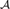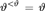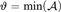Hostname: page-component-7dc689bd49-6fmns Total loading time: 0 Render date: 2023-03-20T20:35:25.486Z Has data issue: true Feature Flags: { "useRatesEcommerce": false } hasContentIssue true

Published online by Cambridge University Press:  22 July 2015

## Abstract

The mad spectrum is the set of all cardinalities of infinite maximal almost disjoint families on ω. We treat the problem to characterize those sets${\rm {\cal A}}$ which, in some forcing extension of the universe, can be the mad spectrum. We give a complete solution to this problem under the assumption$\vartheta ^{ < \vartheta } = \vartheta$, where$\vartheta = {\rm{min}}\left( {\rm {\cal A}} \right)$.

## Keywords

Type
Articles
Information
The Journal of Symbolic Logic , September 2015 , pp. 901 - 916
Shelah, Saharon, Two cardinal invariants of the continuum$\left( {{\rm } < {\rm }} \right)$and fs linearly ordered iterated forcing. Acta Mathematica, vol. 192 (2004), pp. 187233.CrossRefGoogle Scholar